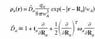# Help interpreting a differential transpose

moller07
Hi, I'm currently trying to decipher an equation in a paper I'm reading for research of my own. However, I am running to a little trouble interpreting their notation and was hoping some of the knowledgeable people on this forum might be able to help. I have attached the image containing the operator and the equation it operates on.

A little background on the equations: The equation it operates on is an electron density distribution, where q and w_a are constants. The variable r is the vector valued position at which you want to evaluate the density, and the variable R_a is the vector valued position of the nucleus. In the operator, the term t_a is a row vector representative of a dipole moment and the term w_a (or omega_a as it's properly written) is a 3x3 tensor.

How exactly this operator operates on the function is a little bit of a mystery to me, especially the part containing the differential transpose. Thus far, I have reasoned that the partial differential with respect to R_a of the function must yield a column vector, of which the components would be the obvious x, y, and z parts. This allows the product between t_a and this vector to once again yield a functional, which is an absolute requirement as having a matrix in my electron density functional would have no well defined meaning.

From this I can reason that in the 3rd term of the operator (counting 1 as the first), that starting from the right, I take the first partial differential and receive a column vector. I then operate with the 3x3 matrix and receive back another column vector. But then I reach the mystery operator, this differential transpose. Somehow by acting this operator on my column vector, I must get back a functional that is of use to me, and I have no idea how to do this.

I have been scouring math texts and the internet for help on this issue, and the closest information I've been finding is in subfields of matrix calculus, but even those have not really been of help to me. I am hoping that someone here might be knowledgeable and kind enough to help me. Thanks in advance.

(The equation and operator are attached as a jpeg image)

#### Attachments

•operator.jpg
3.8 KB · Views: 356

Homework Helper
Writing it in index notation, I think it looks like this (dropping the a subscript so as not to obscure the indices):

$$\hat{D} = 1 + t_j\partial_j + \frac{1}{2}\partial_i\omega_{ij}\partial_j$$.

Here, $\partial_j = \frac{\partial}{\partial R_j}$. Are you familiar with index notation?

If you don't know index notation but you do know vector calculus, then the following explanation probably makes more sense:

Basically, the second term could be interpreted as a dot product $\mathbf{t}\cdot\nabla$ where t is a column vector and $\nabla$ is the gradient operator.

The last term could be interpreted as $\nabla \cdot (\tilde{\omega}\nabla)$, where $\tilde{\omega}$ is the matrix representing omega and $\nabla$ is again the gradient operator which acts on the function in the first line of your attached equation to give a vector, which then multiplies the matrix omega to give another vector. You then take the divergence of the resulting vector.

Last edited: